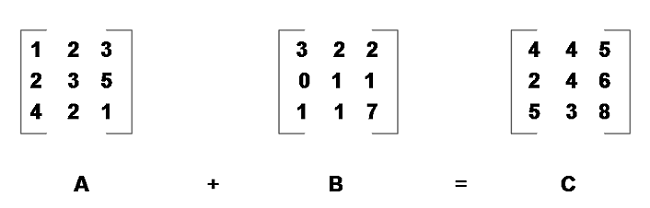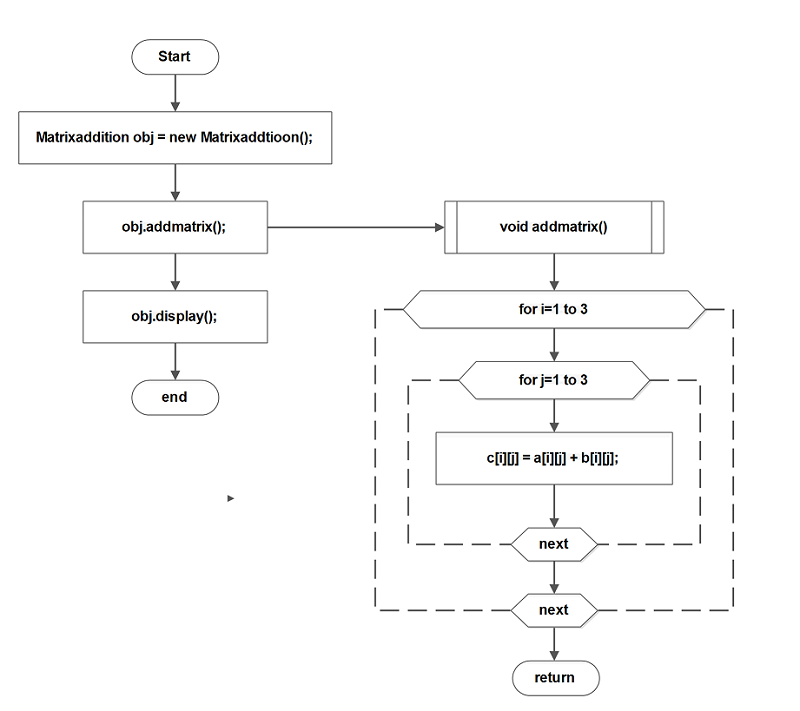## Program in Java for Addition of Two Matrices

The program for addition of two matrices uses simple arithmetic operations on two given matrices and prints the output.

It is written in NetBeans 8.2 installed on a windows 7 64-bit system. You may also need to install the Java SDK version suitable for NetBeans 8.2.

This program is a simple demonstration of arithmetic operations and it is intended for beginner level learner of Java programming.

## Problem Definition

The program reads two matrices – matrix A and matrix B and adds each corresponding element of the given matrix. The result of this addition is stored in a third matrix C.

For example, let A, B, and C be 3 x 3 matrices. ThenIn the above figure, each element from first row of A is added to each element of the B and the sum is stored in the matrix C.

## Flowchart## Program Code

``````/* To change this license header, choose License Headers in Project Properties.
* To change this template file, choose Tools | Templates
* and open the template in the editor.
*/

package JavaExamples;

/**
*
*/

int a[][] = {{1,2,3},{2,3,4},{1,4,5}};

int b[][] = {{1,2,4},{2,3,6},{3,1,5}};

int c[][] = new int ;

int i,j;
for(i=0;i< 3;i++){

for(j=0;j < 3;j++){

c[i][j] = a[i][j] + b[i][j];
}
}
}

void display(){

int i,j;

for(i=0;i < 3;i++){

for(j=0;j < 3;j++){

System.out.print (c[i][j]);

}
System.out.println("n");
}
}

public static void main(String[] args)
{

``````4      4      5